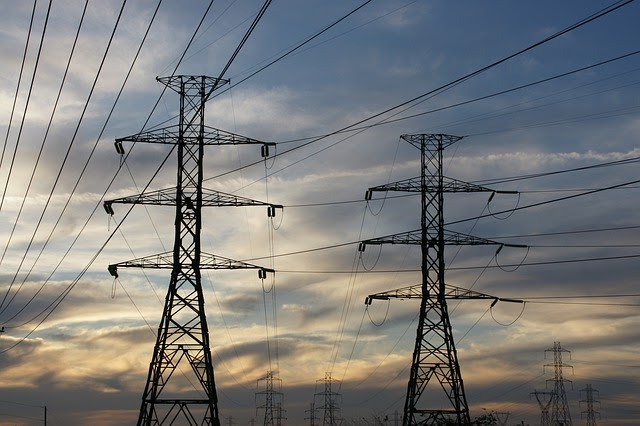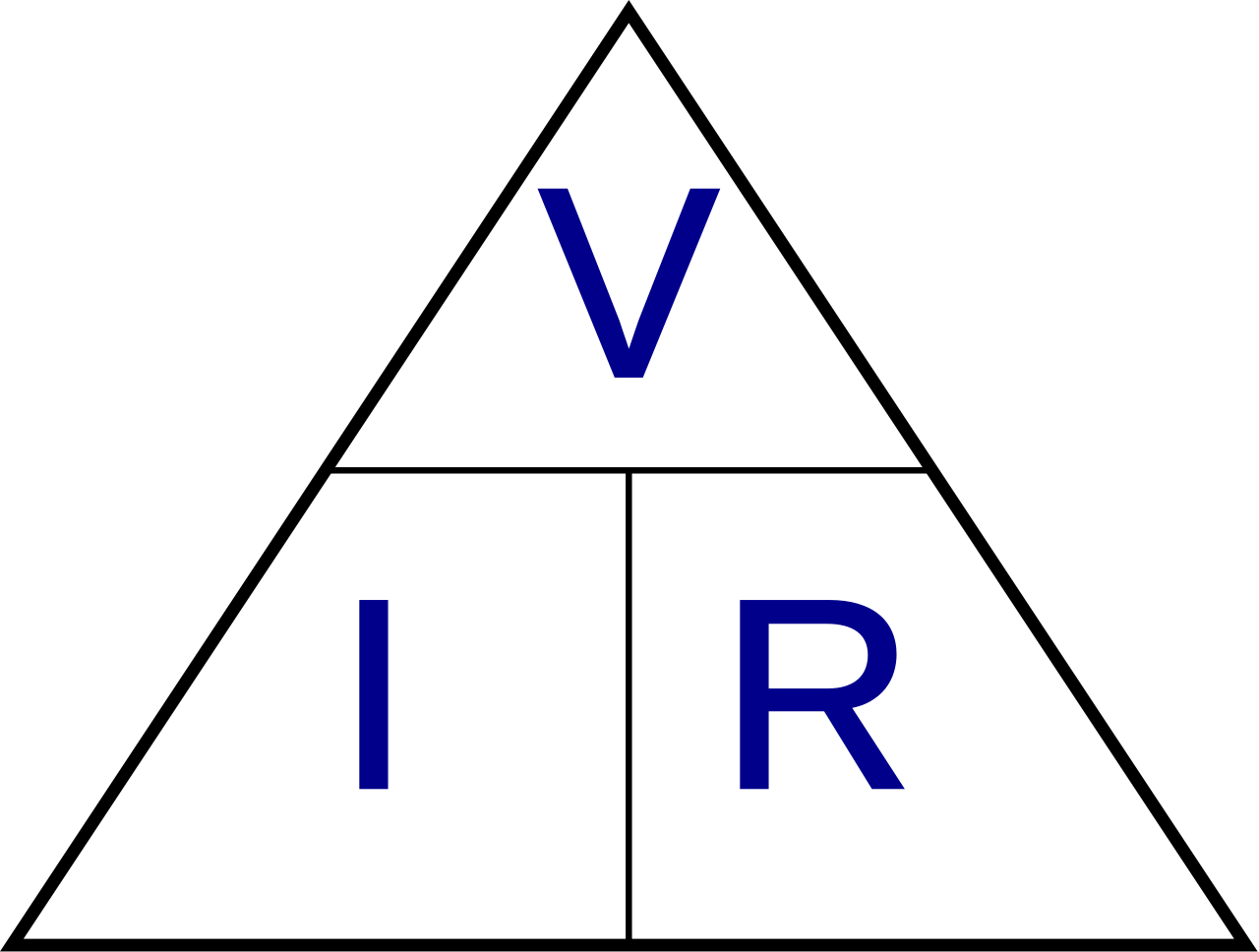Everything worth measuring has a unit of measurement associated with it. In the US, we use inches and feet to measure how tall something is, pounds and ounces to measure how much something weighs and Fahrenheit to measure the temperature of something. But what about electricity? What units of measurement or used to talk about electricity?Before we talk about how to measure electricity, we first need to understand what it is. At a base level, electricity is the movement of electrons. Your computer, your lights, your television, your fridge, etc all operate using the same basic power source - the movement of electrons.

Whenever we talk about the power of electricity, we’re actually talking about the charge created by moving electrons.

The basic units of measurement for electricity are current, voltage and resistance.

## Current (I)

Current, measured in amps, is the rate at which charge is flowing - how fast the electrons are moving. Amps, or amperes, are the basic unit for measuring electricity and measure how many electrons move past a point every second. One amp is equal to 6.25 x 1018 electrons per second.

## Voltage (V)

Voltage, measured in volts, is the difference in charge between two points. Put simply, it is the difference in the concentration of electrons between two points.

## Resistance (R)

Resistance is a material’s tendency to resist the flow of charge (current). It is measured in ohms.

## The Water Pipe Analogy

Now, let’s put these ideas to work. The most common analogy used to understand these ideas is water in a pipe. When thinking about how fast water can move through a pipe, there are three main components that need to be considered: water pressure, flow rate and pipe size. To combine these two ideas, voltage is equivalent to water pressure, current is the flow rate and resistance is the pipe size.

So, when we talk about these values, we’re really describing the movement of charge, and thus, the behavior of electrons. A circuit is a closed loop that allows charge to move from one place to another. Components in the circuit allow us to control this charge and use it to do work.

## Ohm’s Law

Ohm’s Law is a basic, and highly important, equation that is used to determine how current, voltage and resistance interact. It says that the current is equal to the voltage divided by the resistance or I = V/R. Ohm’s Law can be used to accurately describe the conductivity of most electrically conductive materials. As long as you know two of the values, it is possible to determine the third. The three variations of this equation are: I = V/R, V=IR, R=V/I## Watt

There is another term you may have heard used in relation to electricity: Watts. Watts measure the rate at which energy is used or transferred and are not just used for electronics. A Watt is the basic unit of electric, mechanical, or thermal power. One Watt is equal to one amp under the pressure of one volt. (Watt = Amps x Volts)

For a more in-depth look at voltage, current, resistance, & Ohm's Law, check out this post.